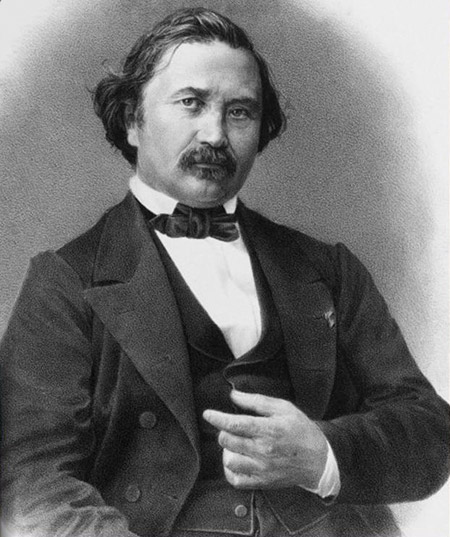A# Bertrand's postulateJoseph Bertrand.

Bertrand's postulate, also known as Bertrand's conjecture, states that if n is an integer greater than 3, then there is at least one prime number between n and 2n-2. This postulate (which should now be called a theorem) is named after the French mathematician Joseph Bertrand (1822–1900) who, in 1845, showed it was true for values of n up to 3,000,000. The Russian Pafnuty Chebyshev (1821–1894) gave the first complete proof in 1850, so that it is sometimes called Chebyshev's theorem (although another theorem also goes by this name). In 1932 Paul Erdös gave a more elegant proof, using the binomial coefficients, which is the one that appears in most modern textbooks. Bertrand's postulate implies that the n-th prime pn is at most 2n.